RS Aggarwal Class 7 Solutions Chapter 18 - Reflection And Rotational Symmetry

RS Aggarwal Class 7 Chapter 18 - Reflection And Rotational Symmetry Solutions Free PDF

The reflection symmetry is one of the simplest forms of symmetry which deals with the reflection of objects. A figure which exhibits reflectional symmetry does not change, when it undergoes reflectional symmetry. It is also referred to as by other names such as line symmetry, mirror symmetry and mirror image symmetry.

Radial symmetry which is also commonly known as rotational symmetry is defined as the property of an object which looks the same after the rotation by a small partial turn. The degree of rotational symmetry can be defined by the number of distinct orientations.

Check out the RS Aggarwal Class 7 Solutions Chapter 18 Rotational Symmetry below: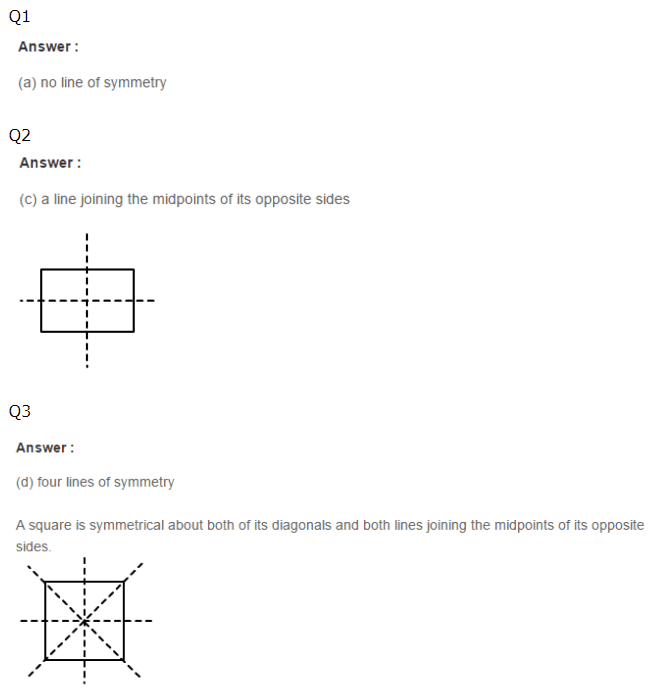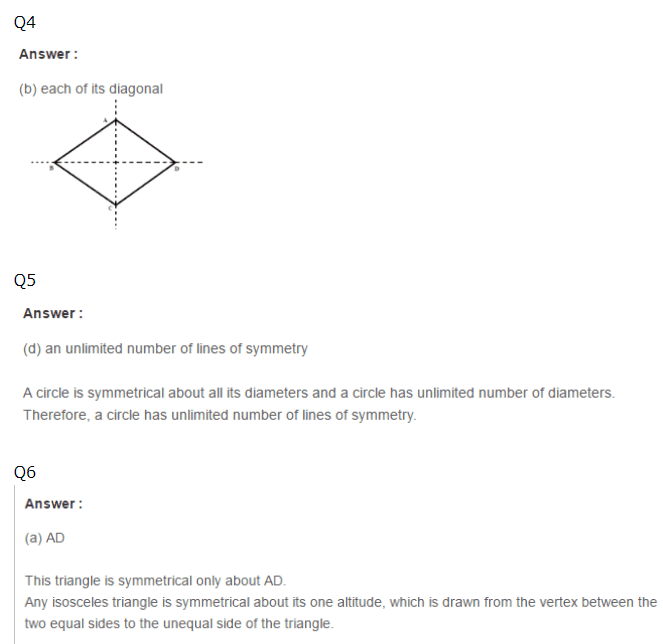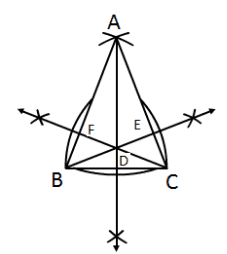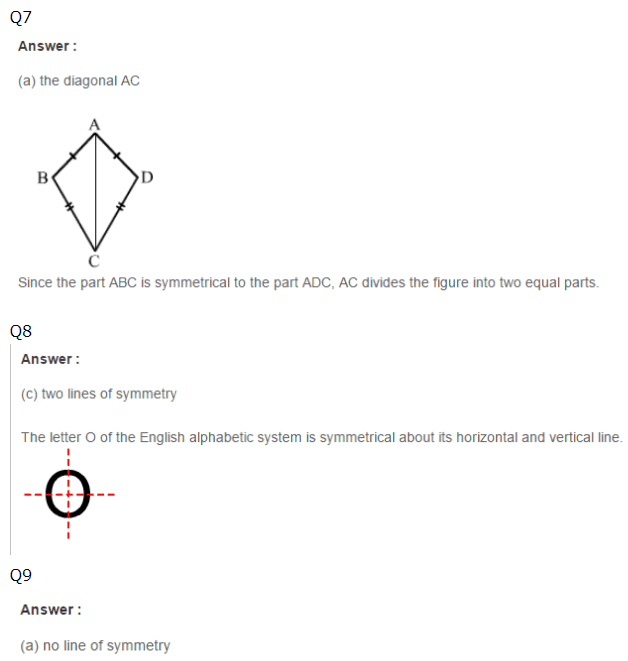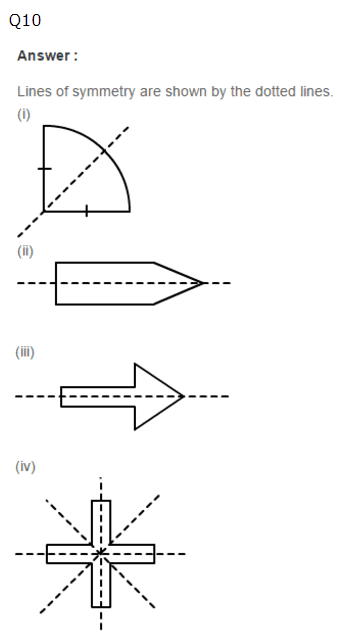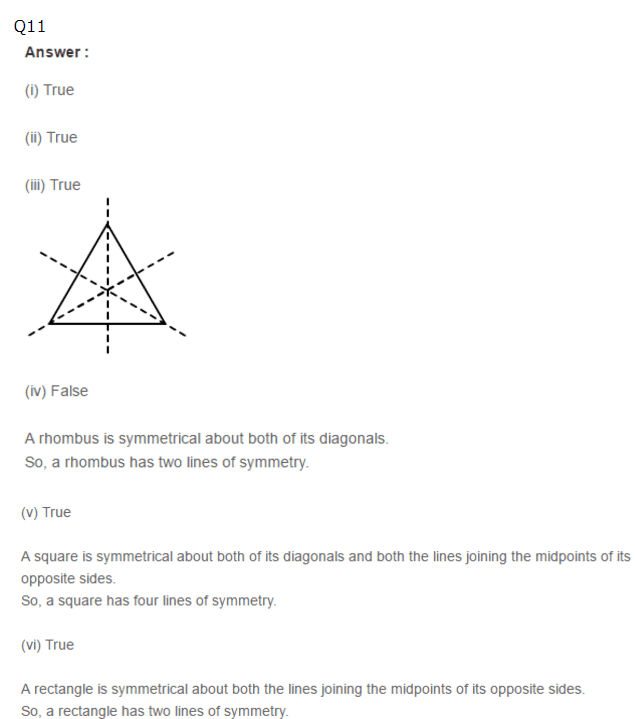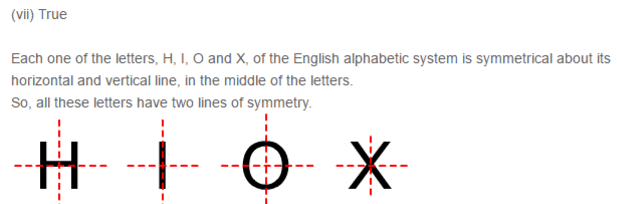Practise This Question

Can this net diagram be used for a die? (True/False)